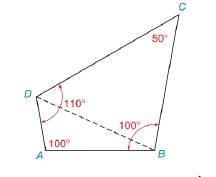Chapter 3.5, Problem 3E### Elementary Geometry for College St...

6th Edition
Daniel C. Alexander + 1 other
ISBN: 9781285195698

#### Solutions

Chapter
Section### Elementary Geometry for College St...

6th Edition
Daniel C. Alexander + 1 other
ISBN: 9781285195698
Textbook Problem
42 views

# In Exercise 1 to 10, classify each statement as true or false. D B > A BTo determine

Whether the statement is true or false.

Explanation

Given:

The following figure shows the given diagram.

Given that,

DB>AB.

Approach:

If the measure of one angle of a triangle is greater than the measure of a second angle, then the side opposite the larger angle is longer than the side opposite the smaller angle.

As shown in the above figure, the measure of A is larger than the measure of B

### Still sussing out bartleby?

Check out a sample textbook solution.

See a sample solution

#### The Solution to Your Study Problems

Bartleby provides explanations to thousands of textbook problems written by our experts, many with advanced degrees!

Get Started

#### Evaluate the integrals in Problems 7-18. 17.

Mathematical Applications for the Management, Life, and Social Sciences

#### Simplify the expressions in Exercises 97106. 32/331/6

Finite Mathematics and Applied Calculus (MindTap Course List)

#### The polar coordinates of the point P in the figure at the right are:

Study Guide for Stewart's Single Variable Calculus: Early Transcendentals, 8th